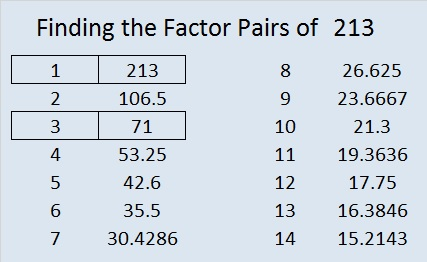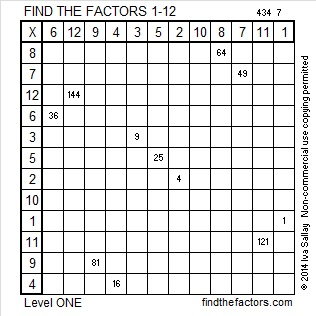# 213 and Level 1

• 213 is a composite number.
• Prime factorization: 213 = 3 x 71
• The exponents in the prime factorization are 1 and 1. Adding one to each and multiplying we get (1 + 1)(1 + 1) = 2 x 2 = 4. Therefore 213 has 4 factors.
• Factors of 213: 1, 3, 71, 213
• Factor pairs: 213 = 1 x 213 or 3 x 71
• Since 213 has no square factors, the square root of 213 cannot be simplified. √213 ≈ 14.595I recommend reading a-math-book-to-change-your-teaching, an article written by a fellow blogger about a book that simplifies the teaching of mathematics while making the subject more meaningful for students.Excel file of puzzles and previous week’s factor solutions: 12 Factors 2014-08-25